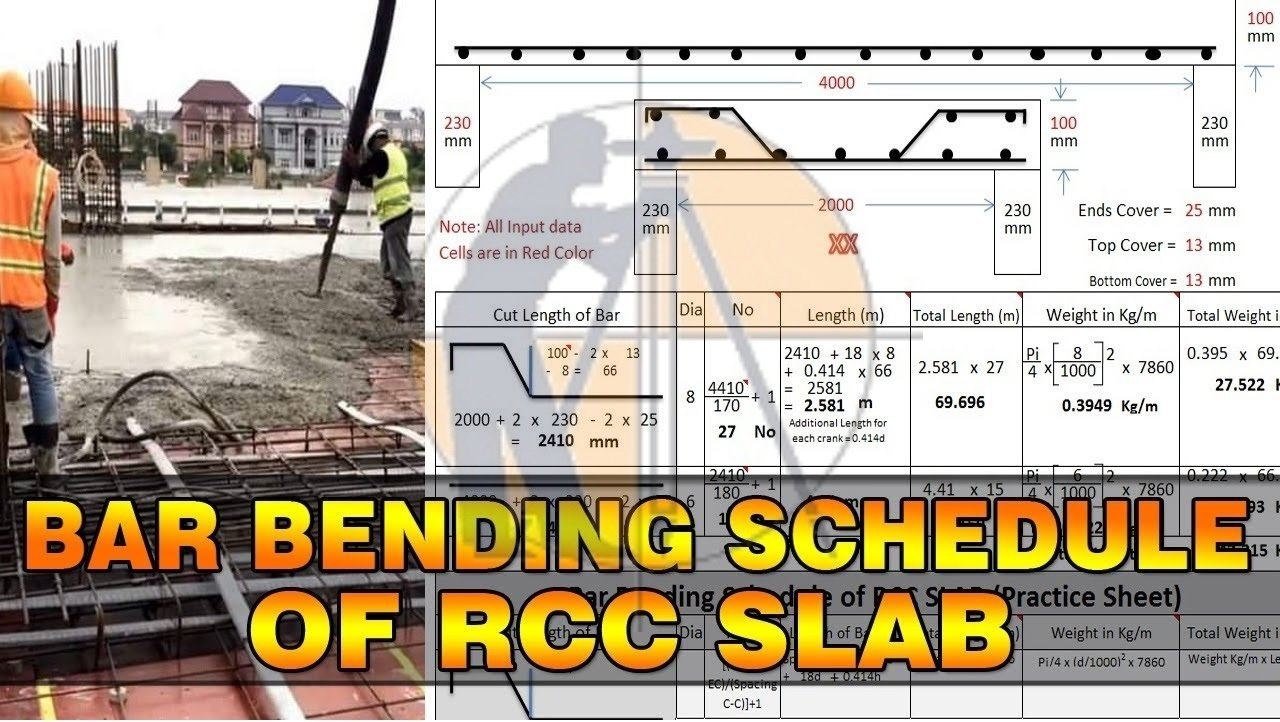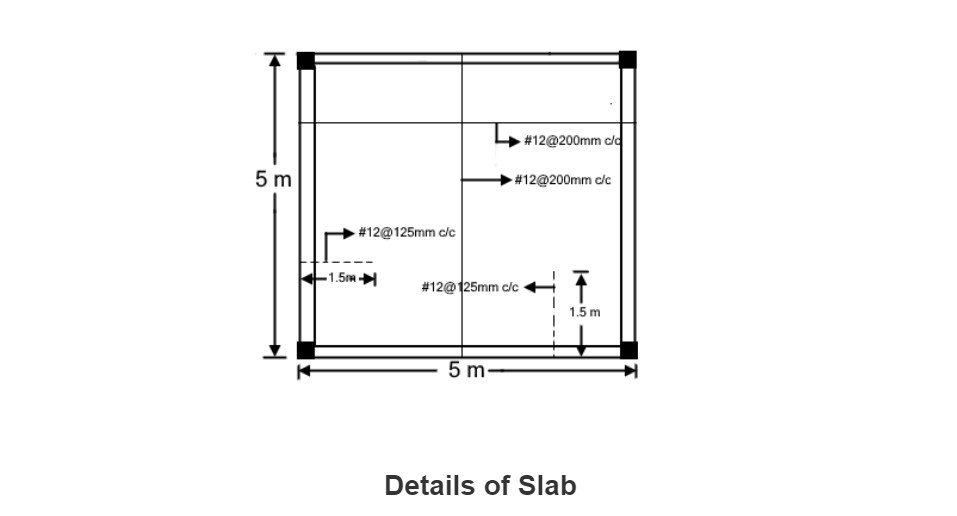Breaking News

# BBS for RCC Slab

## Bar Bending Schedule for RCC Slab.

To preparing BBS for a Slab. there are some criteria and assumptions that require to be met Let’s get started.### Forces on Slab

U should know the normal forces action on a Slab.

If you see the shear force and bending moment diagram, the slab has high moments at center and less at ends. The shear force is maximum at the ends while at the center shear force is zero or minimum.

• Use less cutting of steel and provide desirable lapping.
• Lap another steels at same time.
• Don’t Overlap at the same position/ place.
• Between the laps end to end spacing should be provided.

### BBS Used by

BBS is used by different kind of peoples and officials on construction site which is given below:

• Quantity surveyor
• Steel fixer
• Contractor
• Project manager
• Inspectors
• Clerks of construction

In Construction site all estimation and costing operation is the responsibilities of Quantity Surveyor. The B.B.S gives the details to Quantity Surveyor for finding out the number of steel its type, size and shape. Contractor are using B.B.S for ordering the steel bar for proceeding the construction. Steel fixer using B.B.S for doing their work or installing the bar in Column, Beam or Slab according to the B.B.S that how much cut length or bending of bar will be needed and which size of steel should be use. The inspector & clerks of construction refers to the B.B.S to make are that the reinforcement work in the field is in proper with the design reinforcement as per drawing.

## Bar Bending Schedule for Slab#### Details from the diagram:

1. Thickness of slab is150 mm
2. Development Length is 50d
3. Bottom bar of slab will be provided 12 mm dia of steel is at 200 mm center to center spacing
4. Top bar of slab will be provided 12 mm dia of steel is at 200 mm center to center spacing
5. Extra bar of slab at x- axis will be provided 12 mm dia of steel is at 125 mm center to center spacing
6. Extra bar of slab at y- axis will be provided 12 mm dia of steel is at 125 mm center to center spacing
7. Stirrup spacing is 8mm @ 150 mm C/C
8. Concrete Cover of slab is 25 mm

### Steps for Bar Bending Schedule of Slab

Generally, there are five steps for preparing the B.B.S of column these five is given below:

1. Number of steel
2. Cut length of steel
3. Find out lapping
4. B.B.S

### Numbers of steel in Main bar

Number of steels = (Total length of slab ÷ spacing of bar) +1

Number of stirrups = 5000 ÷ 200 + 1

Number of stirrups = 26 numbers

### Numbers of steel in Distribution bar

Number of steels = (Total length of slab ÷ spacing of bar) +1

Number of stirrups = 5000 ÷ 200 + 1

Number of stirrups = 26 numbers

### Numbers of Extra bar at x-axis

Number of steels = (Total length of slab ÷ spacing of bar) +1

Number of stirrups = 5000 ÷ 125 + 1

Number of stirrups = 41 numbers

### Numbers of Extra bar at y-axis

Number of steels = (Total length of slab ÷ spacing of bar) +1

Number of stirrups = 5000 ÷ 125 + 1

Number of stirrups = 41 numbers

### Cut Length of Main Bar

Length of Main bar = slab length – 2(concrete cover) + Development length (Ld) + Overlap Length

= 5000 – 2(25) + (12×12) + (50×12)

= 5694 mm or 5.69 m

### Now we find the length of bar

The total length of Main bar = 5694 x number of steels in slab

The total length of bar = 5694 x 26

The total length of bar = 148044 mm or 148 meter

### Cut Length of Distribution Bar

Length of Distribution bar = slab length – 2(concrete cover) + Development length (Ld) + Overlap Length

= 5000 – 2(25) + (12×12) + (50×12)

= 5694 mm or 5.69 m

### Now we find the length of bar

The total length of Distribution bar = 5694 x number of steels in slab

The total length of Distribution bar = 5694 x 26

The total length of bar = 148044 mm or 148 meter

### Cut Length of Extra Bar at x-axis

Total Length of extra bar = Length of extra bar – concrete cover

= 1500 – 2(25)

= 1450 mm or 1.450 meter

### Now we find the length of bar

The total length of extra bar at x- axis = 1450 x number of extra bar in slab

The total length of bar = 1450 x 41

The total length of bar = 59450 mm or 59.45 meter

### Cut Length of Extra Bar at y-axis

Length of Extra Bar at y-axis = slab length – 2(concrete cover)

= 1500 – 2(25)

= 1450 mm or 1.40 m

### Now we find the length of bar

The total length of Extra bar = 1450 x number of extra bar in slab

The total length of bar = 1450 x 41

The total length of bar = 59450 mm or 59.45 meter

### Main Bar

length of lapping is 50d

Lapping length = 50 x Dia of steel

Length of lapping = 50×12 = 600 mm.

We know that the length of typical steel is 12.25 m or 40 feet length

Total Length of Main Bar = 148044 m that is more than 12.25 m or 40 feet so lapping should be provided.

Length of lapping is 600 mm the result will be 148044 + 600 = 148644 m

It is mentioned already in this article, consider the points while lapping the bar. There is no one right process we can use. Just remember the lines.

• Don’t tie a column at bottom and also at top
• Lap at another bars every time
• Overlap top bars with bottom bars always

### Distribution Bar

length of lapping is 50d

Lapping length = 50 x Dia of steel

Length of lapping = 50×12 = 600 mm.

We know that the length of typical steel is 12.25 m or 40 feet length

Total Length of Main Bar = 148044 m that is more than 12.25 m or 40 feet so lapping should be provided.

Length of lapping is 600 mm the result will be 148044 + 600 = 148644 m

It is mentioned already in this article, consider the points while lapping the bar. There is no one right process we can use. Just remember the lines.

• Don’t tie a column at bottom and also at top
• Lap at another bars every time
• Overlap top bars with bottom bars always

### Numbers of steel in Main bar

Number of steels = (Total length of slab ÷ spacing of bar) +1

Number of stirrups = 5000 ÷ 200 + 1

Number of stirrups = 26 numbers

### Numbers of steel in Distribution bar

Number of steels = (Total length of slab ÷ spacing of bar) +1

Number of stirrups = 5000 ÷ 200 + 1

Number of stirrups = 26 numbers

### Numbers of Extra bar at x-axis

Number of steels = (Total length of slab ÷ spacing of bar) +1

Number of stirrups = 5000 ÷ 125 + 1

Number of stirrups = 41 numbers

### Numbers of Extra bar at y-axis

Number of steels = (Total length of slab ÷ spacing of bar) +1

Number of stirrups = 5000 ÷ 125 + 1

Number of stirrups = 41 numbers

### Bar Bending Schedule

The B.B.S of column is shown below in table

### THANKS.